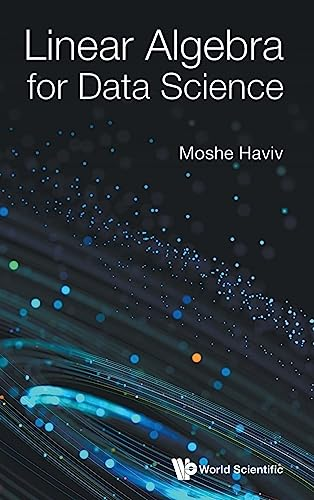# Linear Algebra for Data Science

Description

This book serves as an introduction to linear algebra for undergraduate students in data science, statistics, computer science, economics, and engineering. The book presents all the essentials in rigorous (proof-based) manner, describes the intuition behind the results, while discussing some applications to data science along the way.The book comes with two parts, one on vectors, the other on matrices. The former consists of four chapters: vector algebra, linear independence and linear subspaces, orthonormal bases and the Gram-Schmidt process, linear functions. The latter comes with eight chapters: matrices and matrix operations, invertible matrices and matrix inversion, projections and regression, determinants, eigensystems and diagonalizability, symmetric matrices, singular value decomposition, and stochastic matrices. The book ends with the solution of exercises which appear throughout its twelve chapters.